# Solving Equations by by Solving Equations 1 2

• Slides: 27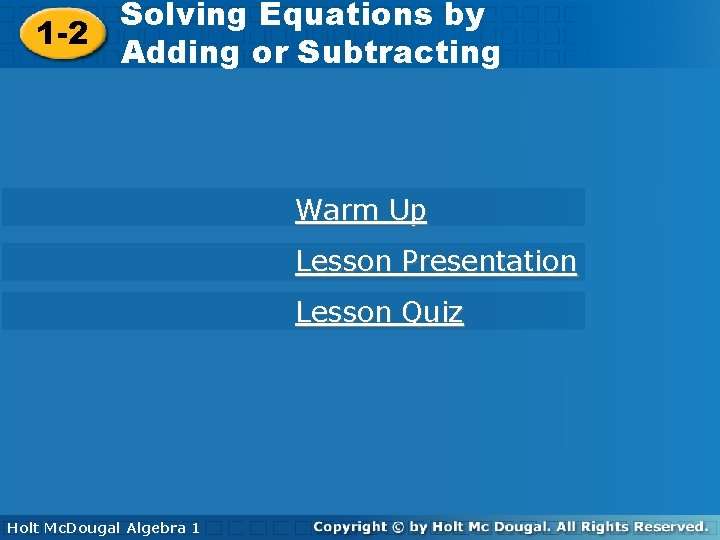Solving Equations by by Solving Equations 1 -2 Adding or Subtracting Warm Up Lesson Presentation Lesson Quiz Holt Algebra Mc. Dougal 1 Algebra 1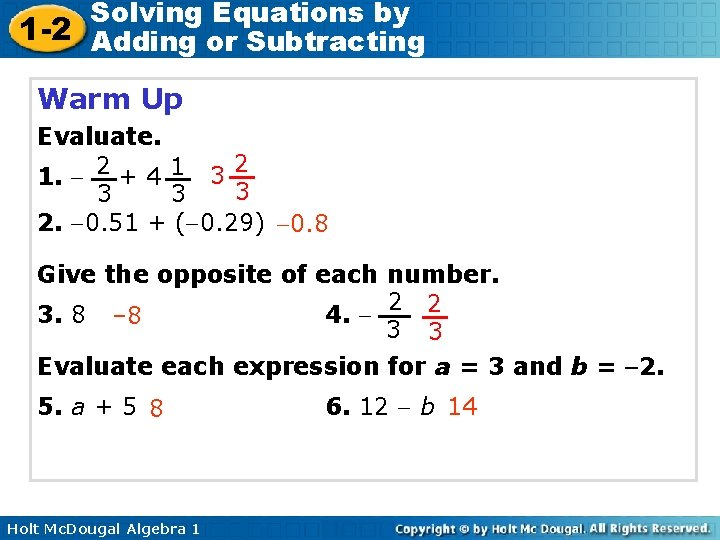Solving Equations by 1 -2 Adding or Subtracting Warm Up Evaluate. 2 1. 2 + 4 1 3 3 2. 0. 51 + ( 0. 29) 0. 8 Give the opposite of each number. 2 2 3. 8 – 8 4. 3 3 Evaluate each expression for a = 3 and b = 2. 5. a + 5 8 Holt Mc. Dougal Algebra 1 6. 12 b 14Solving Equations by 1 -2 Adding or Subtracting Objective Solve one-step equations in one variable by using addition or subtraction. Holt Mc. Dougal Algebra 1Solving Equations by 1 -2 Adding or Subtracting Vocabulary equation solution of an equation Holt Mc. Dougal Algebra 1Solving Equations by 1 -2 Adding or Subtracting An equation is a mathematical statement that two expressions are equal. A solution of an equation is a value of the variable that makes the equation true. To find solutions, isolate the variable. A variable is isolated when it appears by itself on one side of an equation, and not at all on the other side. Holt Mc. Dougal Algebra 1Solving Equations by 1 -2 Adding or Subtracting Isolate a variable by using inverse operations which "undo" operations on the variable. An equation is like a balanced scale. To keep the balance, perform the same operation on both sides. Inverse Operations Operation Inverse Operation Addition Subtraction Holt Mc. Dougal Algebra 1 AdditionSolving Equations by 1 -2 Adding or Subtracting Example 1 A: Solving Equations by Using Addition Solve the equation. Check your answer. y – 8 = 24 +8 +8 y = 32 Check Since 8 is subtracted from y, add 8 to both sides to undo the subtraction. y – 8 = 24 32 – 8 24 Holt Mc. Dougal Algebra 1 24 24 To check your solution, substitute 32 for y in the original equation.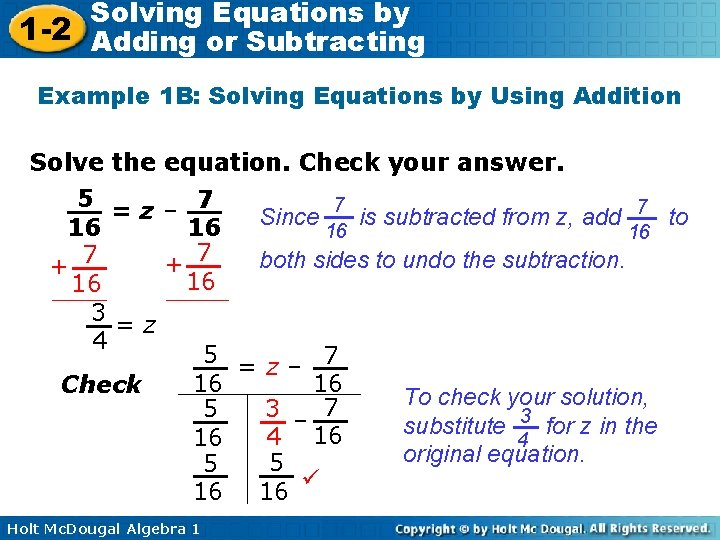Solving Equations by 1 -2 Adding or Subtracting Example 1 B: Solving Equations by Using Addition Solve the equation. Check your answer. 5 =z– 7 7 7 Since is subtracted from z, add to 16 16 both sides to undo the subtraction. + 7 16 16 3=z 4 5 =z– 7 16 16 Check To check your solution, 3 – 7 5 3 substitute for z in the 4 16 16 4 original equation. 5 5 16 16 Holt Mc. Dougal Algebra 1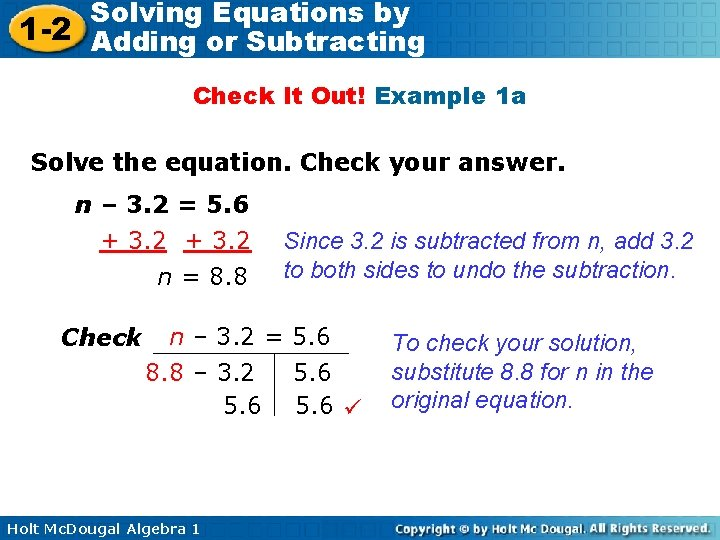Solving Equations by 1 -2 Adding or Subtracting Check It Out! Example 1 a Solve the equation. Check your answer. n – 3. 2 = 5. 6 + 3. 2 n = 8. 8 Check Since 3. 2 is subtracted from n, add 3. 2 to both sides to undo the subtraction. n – 3. 2 = 5. 6 8. 8 – 3. 2 5. 6 Holt Mc. Dougal Algebra 1 5. 6 To check your solution, substitute 8. 8 for n in the original equation.Solving Equations by 1 -2 Adding or Subtracting Check It Out! Example 1 b Solve the equation. Check your answer. – 6 = k – 6 +6 + 6 Since 6 is subtracted from k, add 6 to both sides to undo the subtraction. 0=k Check – 6 = k – 6 – 6 Holt Mc. Dougal Algebra 1 0– 6 To check your solution, substitute 0 for k in the original equation.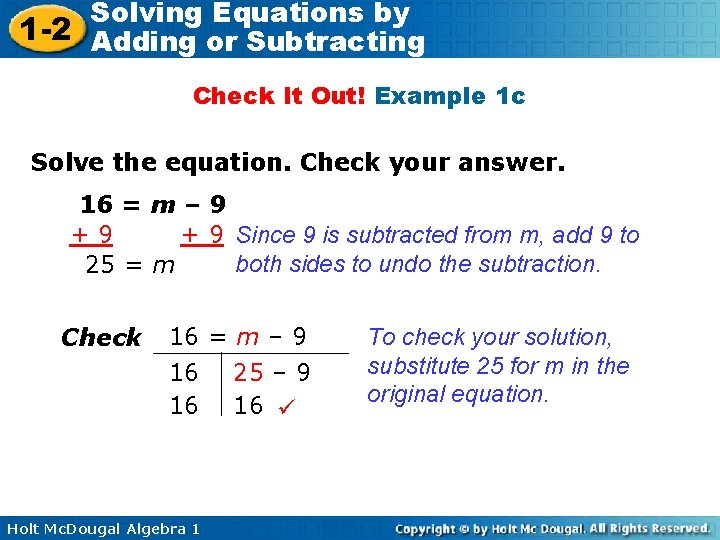Solving Equations by 1 -2 Adding or Subtracting Check It Out! Example 1 c Solve the equation. Check your answer. 16 = m – 9 +9 + 9 Since 9 is subtracted from m, add 9 to both sides to undo the subtraction. 25 = m Check 16 = m – 9 16 16 Holt Mc. Dougal Algebra 1 25 – 9 16 To check your solution, substitute 25 for m in the original equation.Solving Equations by 1 -2 Adding or Subtracting Example 2 A: Solving Equations by Using Subtraction Solve the equation. Check your answer. m + 17 = 33 – 17 Since 17 is added to m, subtract 17 m = 16 from both sides to undo the addition. Check m + 17 = 33 16 + 17 33 Holt Mc. Dougal Algebra 1 33 33 To check your solution, substitute 16 for m in the original equation.Solving Equations by 1 -2 Adding or Subtracting Example 2 B: Solving Equations by Using Subtraction Solve the equation. Check your answer. 4. 2 = t + 1. 8 – 1. 8 2. 4 = t Check Since 1. 8 is added to t, subtract 1. 8 from both sides to undo the addition. 4. 2 = t + 1. 8 4. 2 Holt Mc. Dougal Algebra 1 To check your solution, 2. 4 + 1. 8 substitute 2. 4 for t in the original equation. 4. 2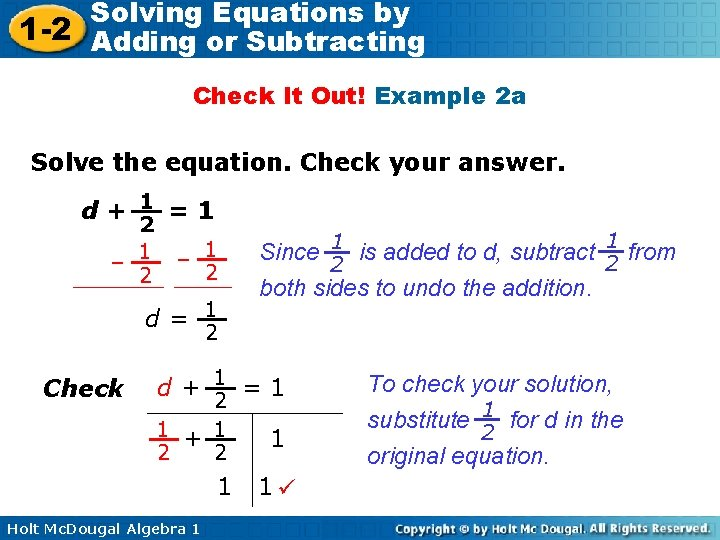Solving Equations by 1 -2 Adding or Subtracting Check It Out! Example 2 a Solve the equation. Check your answer. d+ 1 =1 2 – 1 2 2 d= 1 1 Since 1 is added to d, subtract 2 from 2 both sides to undo the addition. 2 Check d+ 1 =1 2 1 + 1 2 2 1 Holt Mc. Dougal Algebra 1 1 1 To check your solution, substitute 1 for d in the 2 original equation.Solving Equations by 1 -2 Adding or Subtracting Check It Out! Example 2 b Solve the equation. Check your answer. – 5 = k + 5 – 5 Since 5 is added to k, subtract 5 from both sides to undo the subtraction. – 10 = k Check – 5 = k + 5 – 5 Holt Mc. Dougal Algebra 1 – 10 + 5 – 5 To check your solution, substitute – 10 for k in the original equation.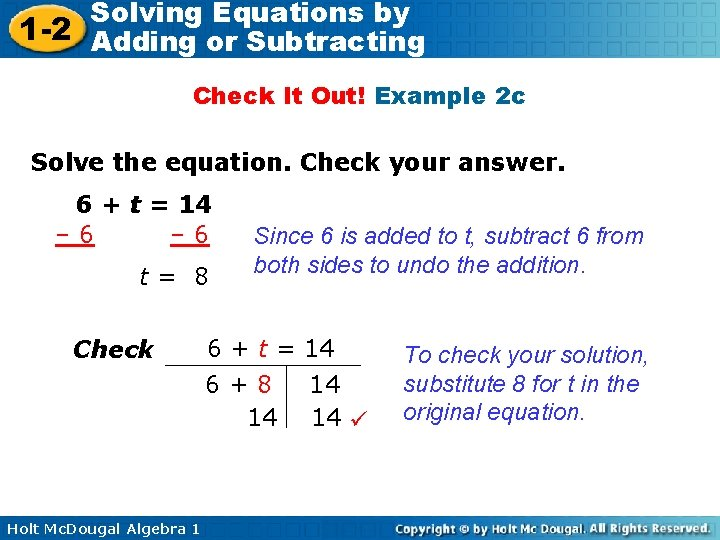Solving Equations by 1 -2 Adding or Subtracting Check It Out! Example 2 c Solve the equation. Check your answer. 6 + t = 14 – 6 t= 8 Check Since 6 is added to t, subtract 6 from both sides to undo the addition. 6 + t = 14 6+8 14 Holt Mc. Dougal Algebra 1 14 14 To check your solution, substitute 8 for t in the original equation.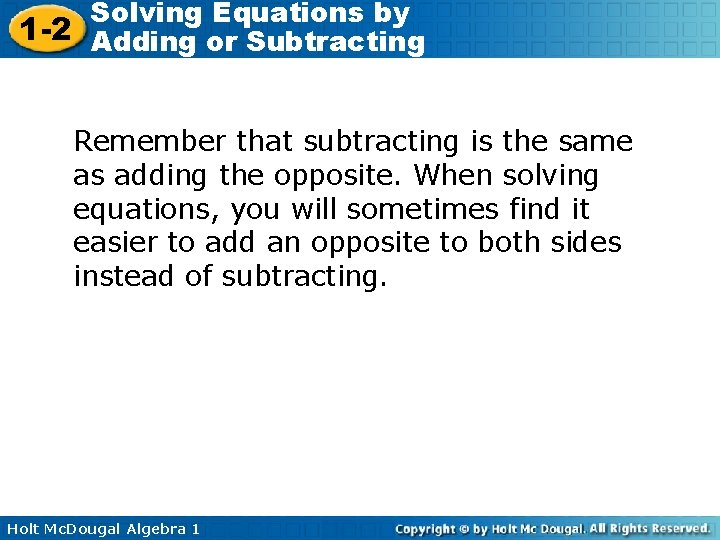Solving Equations by 1 -2 Adding or Subtracting Remember that subtracting is the same as adding the opposite. When solving equations, you will sometimes find it easier to add an opposite to both sides instead of subtracting. Holt Mc. Dougal Algebra 1Solving Equations by 1 -2 Adding or Subtracting Example 3: Solving Equations by Adding the Opposite 2. Solve – 5 + p = – 11 11 + 5 11 11 3 p= 11 Check Since – 5 is added to p, add 5 11 11 to both sides. – 5 +p=– 2 11 11 3 – 2 5 – + 11 11 11 2 2 – – 11 Holt Mc. Dougal Algebra 1 To check your solution, substitute 3 for p in the 11 original equation.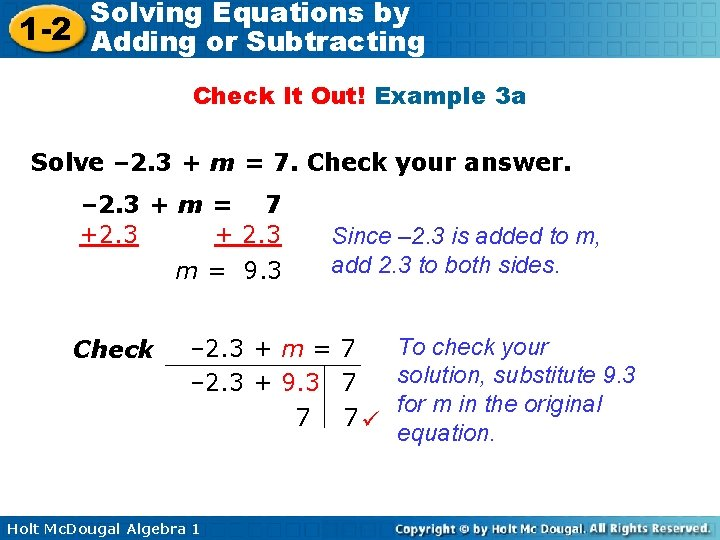Solving Equations by 1 -2 Adding or Subtracting Check It Out! Example 3 a Solve – 2. 3 + m = 7. Check your answer. – 2. 3 + m = 7 +2. 3 + 2. 3 m = 9. 3 Check Since – 2. 3 is added to m, add 2. 3 to both sides. To check your – 2. 3 + m = 7 solution, substitute 9. 3 – 2. 3 + 9. 3 7 for m in the original 7 7 equation. Holt Mc. Dougal Algebra 1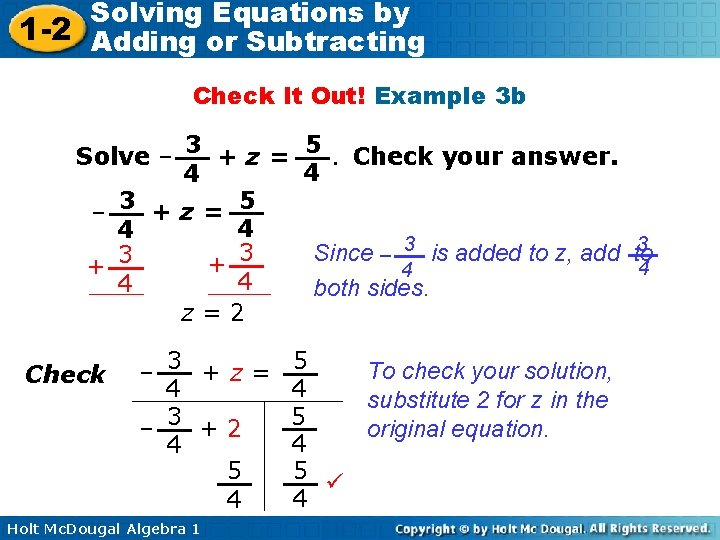Solving Equations by 1 -2 Adding or Subtracting Check It Out! Example 3 b 5. Check your answer. Solve – 3 + z = 4 4 3 +z= 5 – 4 4 3 3 Since – is added to z, add to 3 3 + 4 4 4 both sides. z=2 Check To check your solution, – 3 +z= 5 4 4 substitute 2 for z in the 5 original equation. – 3 +2 4 4 5 5 4 4 Holt Mc. Dougal Algebra 1Solving Equations by 1 -2 Adding or Subtracting Check It Out! Example 3 c Solve – 11 + x = 33. Check your answer. – 11 + x = 33 +11 x = 44 Check Holt Mc. Dougal Algebra 1 Since – 11 is added to x, add 11 to both sides. – 11 + x = 33 – 11 + 44 33 33 33 To check your solution, substitute 44 for x in the original equation.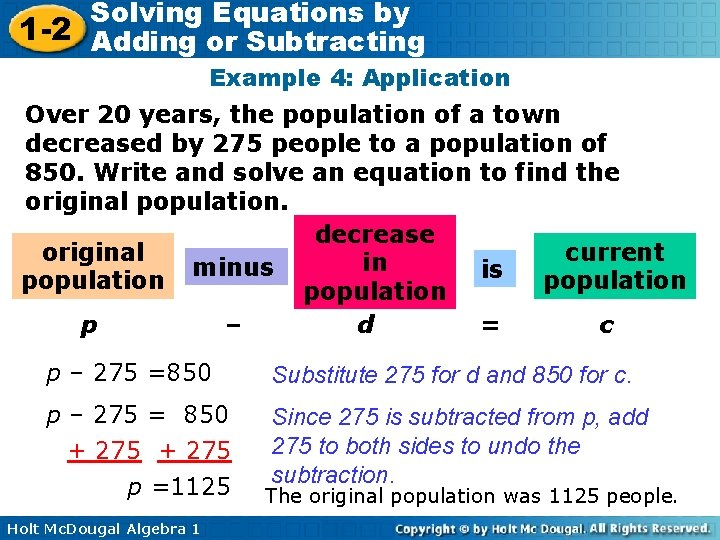Solving Equations by 1 -2 Adding or Subtracting Example 4: Application Over 20 years, the population of a town decreased by 275 people to a population of 850. Write and solve an equation to find the original population. decrease current original in minus is population p – d = c p – 275 =850 Substitute 275 for d and 850 for c. p – 275 = 850 + 275 p =1125 Since 275 is subtracted from p, add 275 to both sides to undo the subtraction. Holt Mc. Dougal Algebra 1 The original population was 1125 people.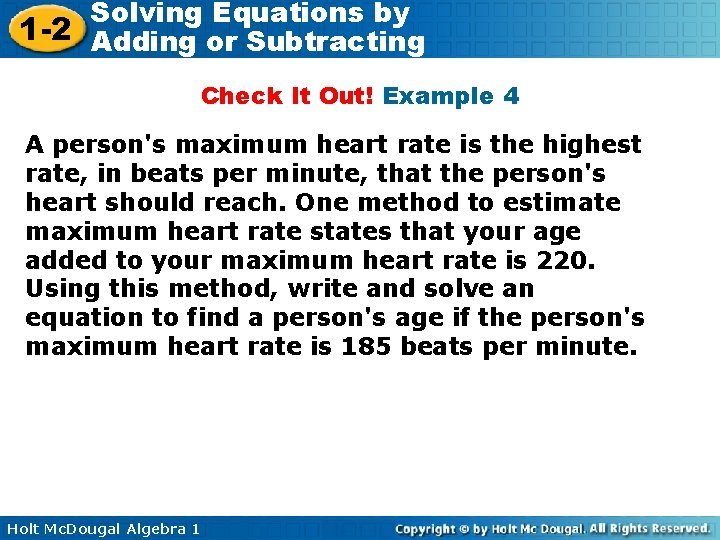Solving Equations by 1 -2 Adding or Subtracting Check It Out! Example 4 A person's maximum heart rate is the highest rate, in beats per minute, that the person's heart should reach. One method to estimate maximum heart rate states that your age added to your maximum heart rate is 220. Using this method, write and solve an equation to find a person's age if the person's maximum heart rate is 185 beats per minute. Holt Mc. Dougal Algebra 1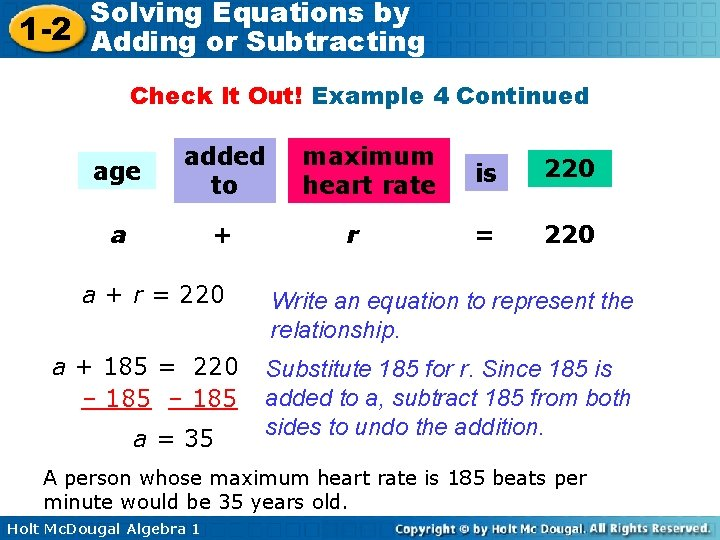Solving Equations by 1 -2 Adding or Subtracting Check It Out! Example 4 Continued age added to a + r = 220 a + 185 = 220 – 185 a = 35 maximum heart rate r is 220 = 220 Write an equation to represent the relationship. Substitute 185 for r. Since 185 is added to a, subtract 185 from both sides to undo the addition. A person whose maximum heart rate is 185 beats per minute would be 35 years old. Holt Mc. Dougal Algebra 1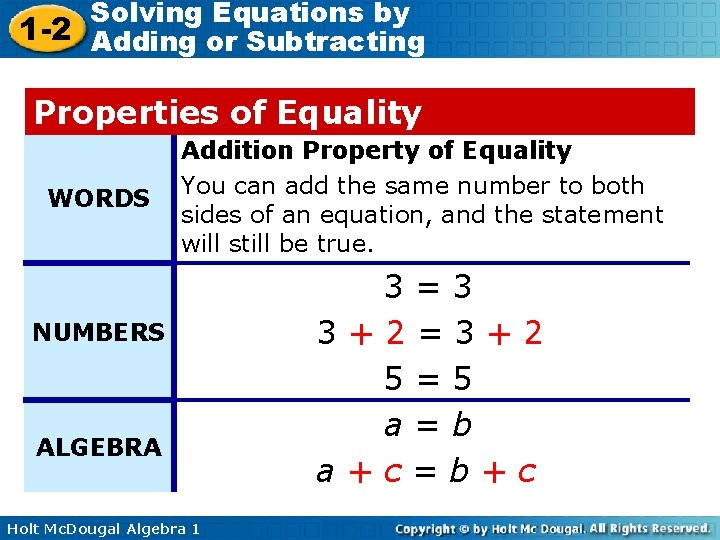Solving Equations by 1 -2 Adding or Subtracting Properties of Equality WORDS Addition Property of Equality You can add the same number to both sides of an equation, and the statement will still be true. NUMBERS ALGEBRA Holt Mc. Dougal Algebra 1 3=3 3+2=3+2 5=5 a=b a+c=b+c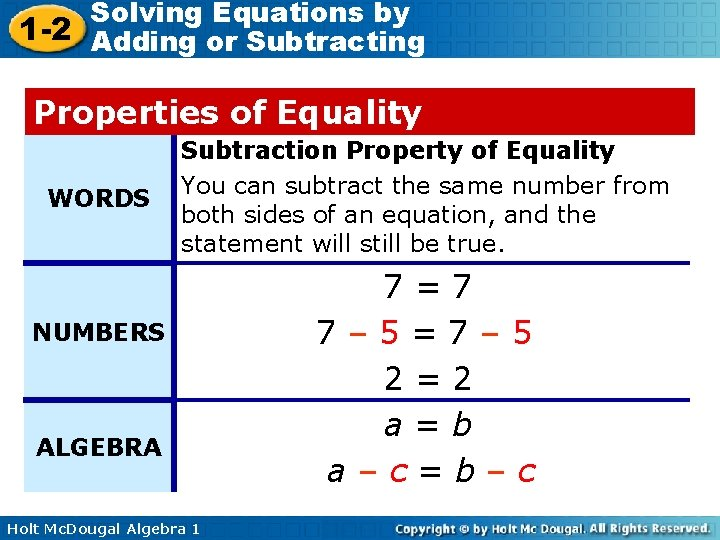Solving Equations by 1 -2 Adding or Subtracting Properties of Equality WORDS Subtraction Property of Equality You can subtract the same number from both sides of an equation, and the statement will still be true. NUMBERS ALGEBRA Holt Mc. Dougal Algebra 1 7=7 7– 5=7– 5 2=2 a=b a–c=b–cSolving Equations by 1 -2 Adding or Subtracting Lesson Quiz Solve each equation. 1. r – 4 = – 8 – 4 2. 3. m + 13 = 58 45 4. 0. 75 = n + 0. 6 0. 15 5. – 5 + c = 22 27 6. This year a high school had 578 sophomores enrolled. This is 89 less than the number enrolled last year. Write and solve an equation to find the number of sophomores enrolled last year. s – 89 = 578; s = 667 Holt Mc. Dougal Algebra 1